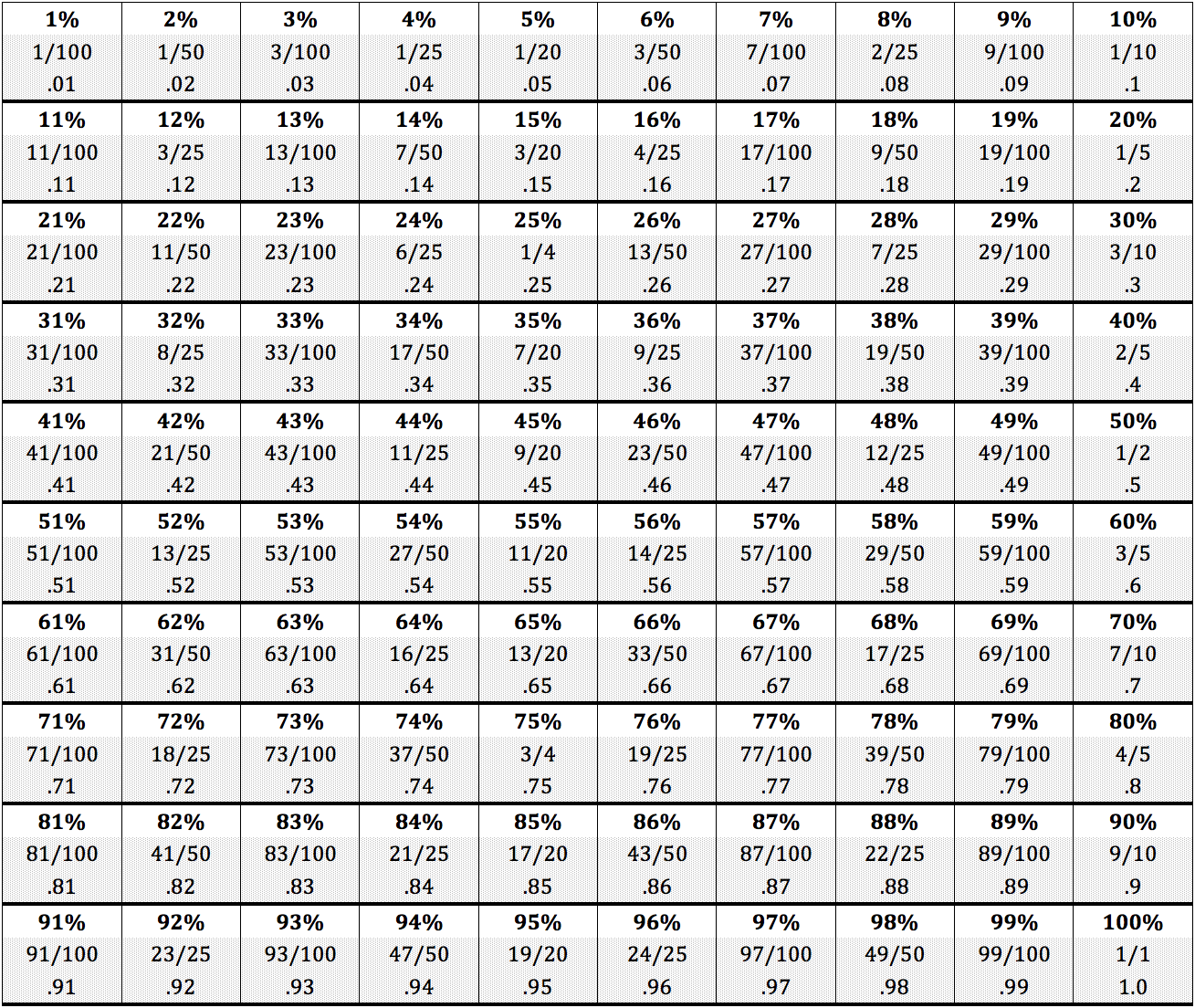A percent is a mathematical relationship between two quantities where one quantity is a particular portion of the other quantity. This relationship is always defined within the context of a certain number of parts out of 100 parts, where the first number is the percentage. You can determine what percent a quantity is of another quantity in two ways: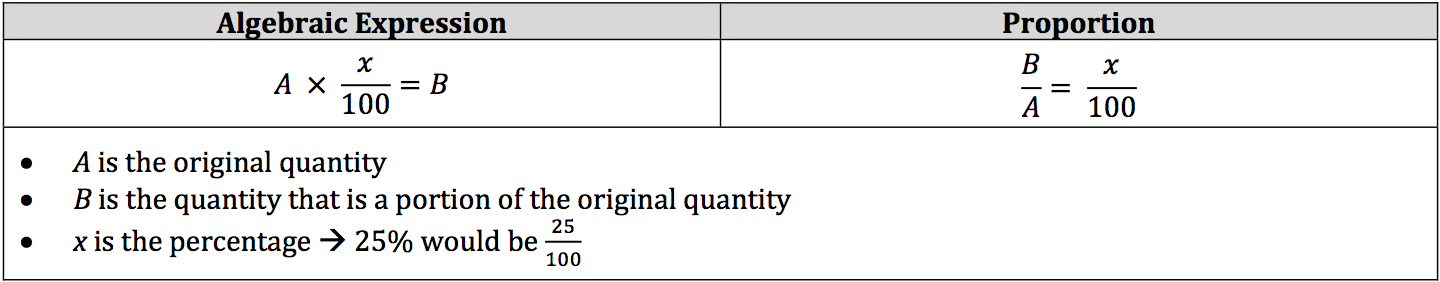Notice that the proportion is actually the same thing as the algebraic expression. The only difference is that the A, or original quantity, switched to the other side of the equals sign by dividing into the B, which is the first step for the algebraic expression when A and B are known quantities. The variable A usually represents a larger number than the variable B.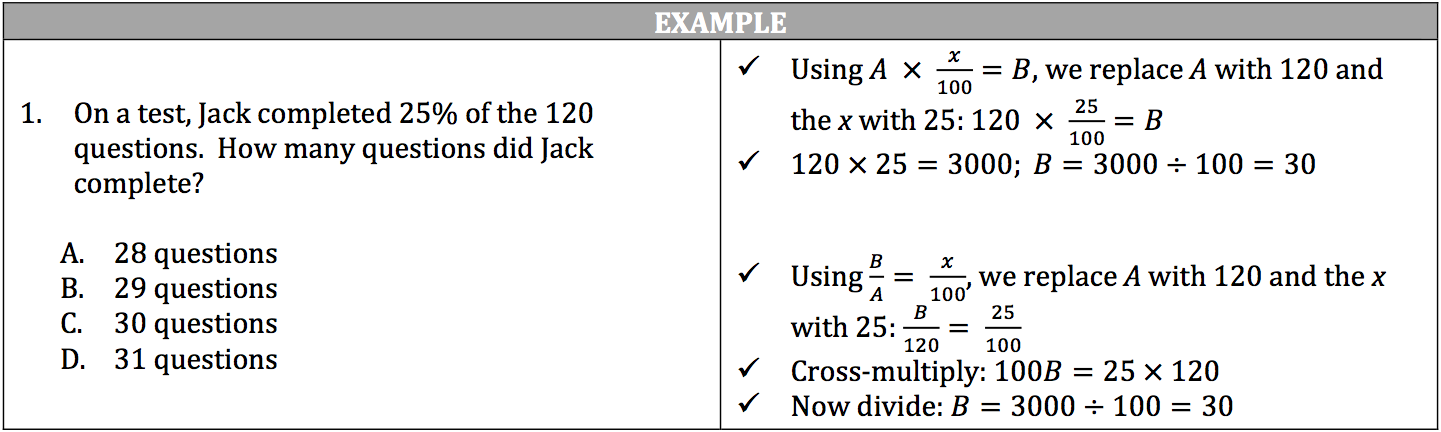Percentages can be represented as a number followed by the % sign, a fraction, or a decimal, but they all mean the same thing.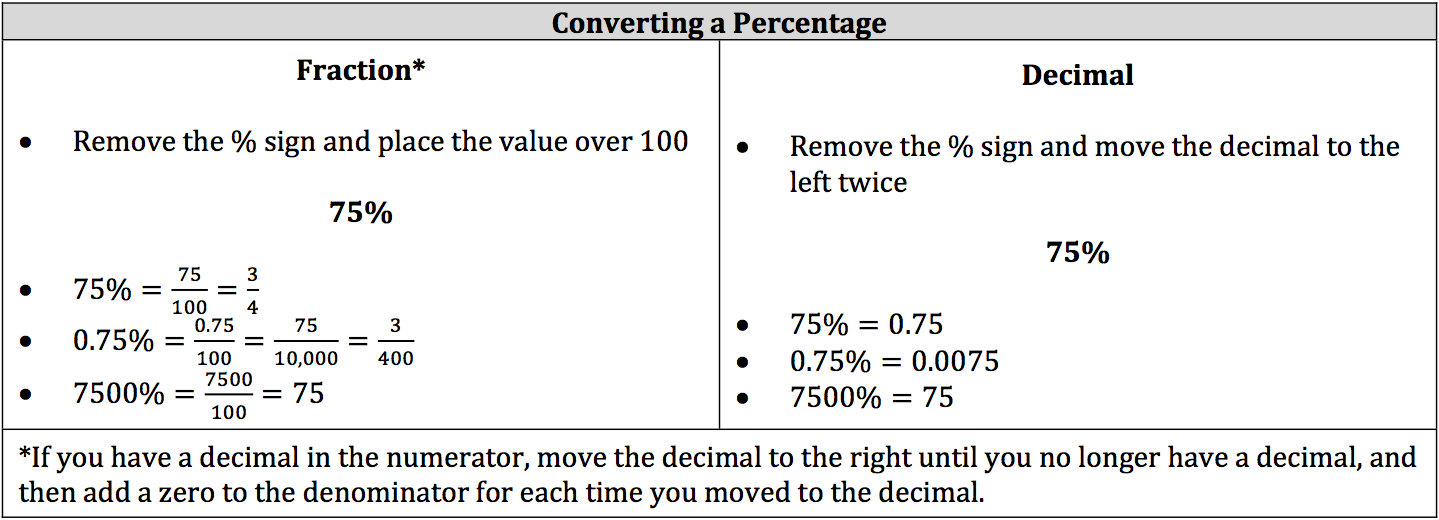In order to increase or decrease a value by a percent, keep these formulas in mind: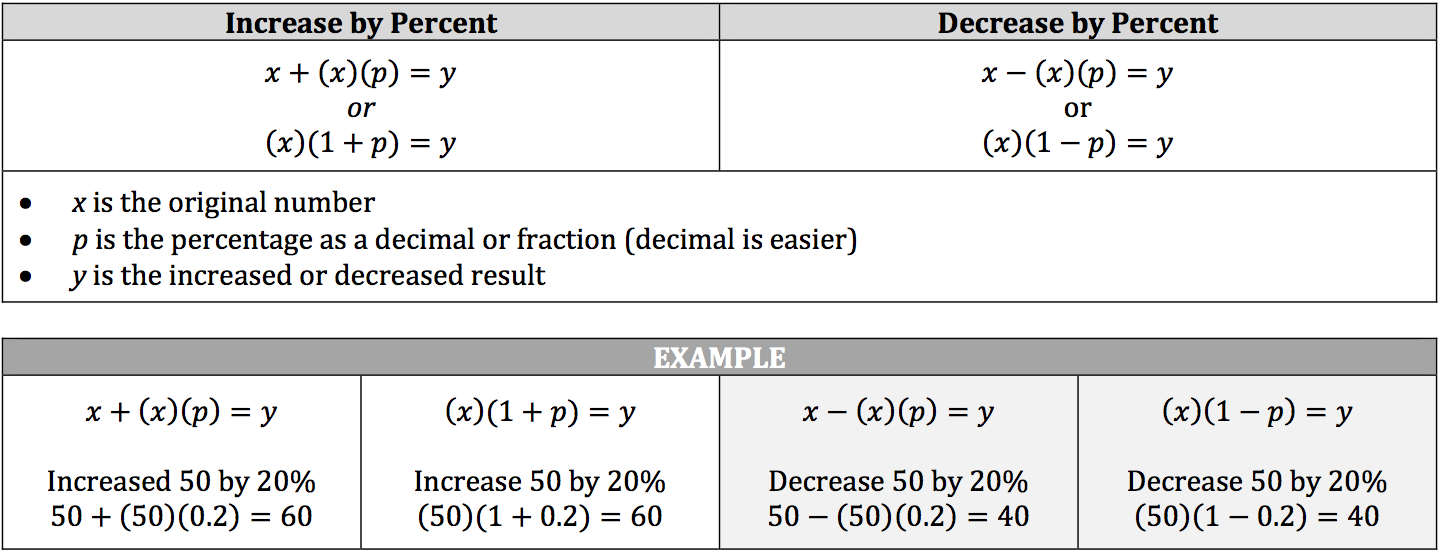Review this chart of percentages, decimals, and reduced fractions from 1% to 100%: